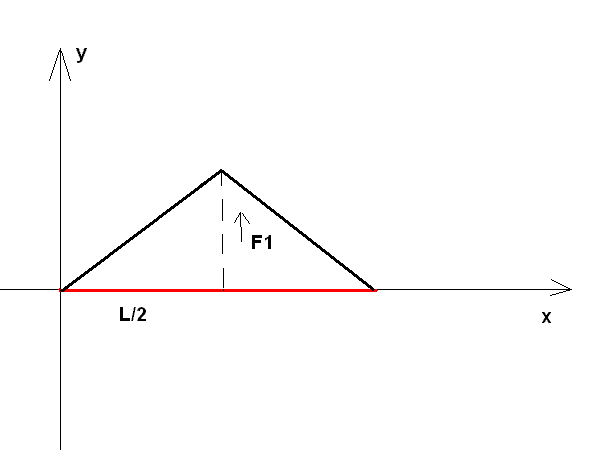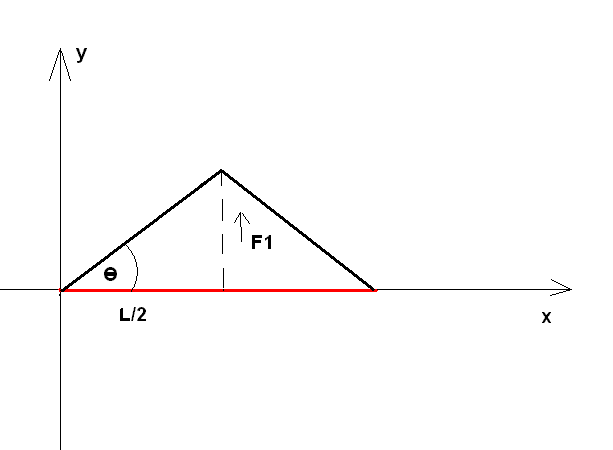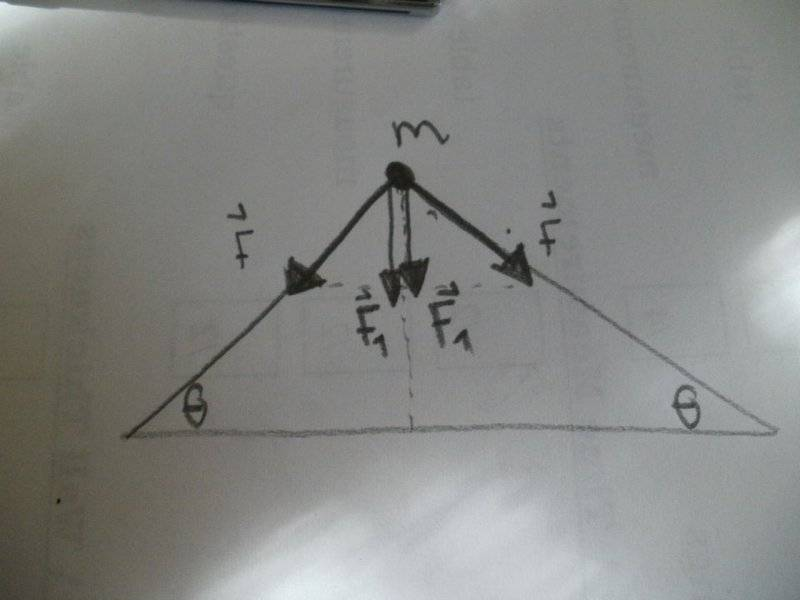# Frequency of wire oscillations

cdummie

## Homework Statement

There's a horizontal thin wire whose mass is negligible and whose length is l=1m. It is strained with constant force F=10N. If we place a tiny pellet in the middle of wire (mass of pellet is m=1g) and then we bring wire out of equilibrium position (moving out of it's original position is normal to the wire, 90 degrees). Determine the frequency of oscillation of wire.

ω=2πf

## The Attempt at a Solution

We know that, since this is oscillation motion, following equation holds:

x'' + ω^2 x=0 ,where x is amplitude, so we could actually try to find acceleration (a=x'') in function of amplitude x, and then, expression multiplied by x would be ω^2 and then, since ω=2πf we could easily find f here, but i don't know how to determine x''. Any ideas?

Mentor
but i don't know how to determine x''.
Draw a diagram with all relevant forces.

Note: That answer works for all mechanics problems where no such diagram has been drawn yet.

•cdummie
Homework Helper
The restoring force is twice the vertical component of the tension in the wire - hope it helps- make a diagram and consider the whole length of the wire

•cdummie
cdummie
Draw a diagram with all relevant forces.

Note: That answer works for all mechanics problems where no such diagram has been drawn yet.This is all i have, i don't know how to draw force F, force F1 is the force that pulls the pellet out of equilibrium position. How can i draw the force that strains wire?

cdummie
The restoring force is twice the vertical component of the tension in the wire - hope it helps- make a diagram and consider the whole length of the wire
I don't understand you very well, why is restoring force (if by restoring force you mean the force that tries to return wire to original position) is twice the component of the tension in the wire?

Homework Helper
Gold Member
There are two tension forces (equal in magnitude but they are not always on the same direction as you can possibly understand) acting on the pellet, one from the half left and one from the half right portion of the wire. You must find the net force (this net force will be the restoring force actually) acting on the pellet and express it in the form k*x where x is the vertical distance from the middle (x is actually the height of the triangle you draw). You might find useful the equality ##cos\theta=\frac{x}{\frac{L}{2}}##

•cdummie
Mentor
This is all i have, i don't know how to draw force F, force F1 is the force that pulls the pellet out of equilibrium position.
It doesn't oscillate as long as you pull it, so you want to consider the case where it is not pulled any more.
How can i draw the force that strains wire?
There is a constant tension of 10 N along the wire. Both sides of the wire pull the mass in the direction of the wire, with a force of 10 N. The two forces are not exactly opposite to each other, which leads to a net force.

•cdummie
Homework Helper
The mass oscillates vertically. The force responsible for these oscillations is directed along along its path,
that is according to Newton's second law the force and acceleration is in the same direction.
This force is supplied by the tension in the string, but only the vertical components of the tension, that
is the components of the tension on both sides contributes.

•cdummie
cdummie
It doesn't oscillate as long as you pull it, so you want to consider the case where it is not pulled any more.There is a constant tension of 10 N along the wire. Both sides of the wire pull the mass in the direction of the wire, with a force of 10 N. The two forces are not exactly opposite to each other, which leads to a net force.

Ok, let's see if i understand this now.This is the same image i posted before, it just has that one angle labeled.

If force that is straining this wire is F and it is acting both from the left and right part of wire, then the net force that is acting is sum of those two forces along the height of the triangle this forces form, right? If that is so, then, since sinθ=F1/F it means that F1=Fsinθ, but there are two F forces so F1=2Fsinθ , but i am still not quite sure about this, since F1 acts in the direction opposite to the direction of the force Fsinθ does it means that force i am looking for is actually F1=-2Fsinθ , is that right?

•Delta2
Homework Helper
Gold Member
What you saying is basically correct but to see if you get it right you must draw the tension forces at the upper vertex of the triangle, the forces are such that the net force is pointing downwards actually. The minus sign comes from the fact that the direction of the net force is always such that it is opposite to the direction of y, where ##sin\theta=\frac{y}{\frac{L}{2}+\epsilon}##. if y is positive (as you draw it) the net force will be pointing downwards, so will be negative, if y is negative the net force will be pointing upwards). Why that happens is because that is just how the wire behaves, if you push it upwards the restoring force will be such as to bring it downwards, if you push it downwards the restoring force will be such as to bring it upwards.

Last edited:
•cdummie
Mentor
does it means that force i am looking for is actually F1=-2Fsinθ , is that right?
Right.
A negative value for F1 is fine, you'll need that sign later when you consider the (now defined as positive) position of the object.

•cdummie
Homework Helper
The two vertical components of the tension in the wire are directed downwards, F1, while the pellet is displaced upwards from the equilibrium position.•cdummie and Delta2